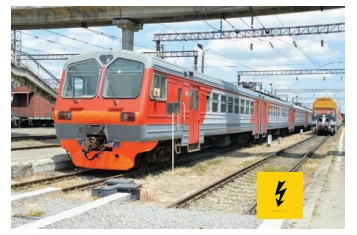Home | | Science 7th Std | Potential difference (v)

# Potential difference (v)

Electrical charges need energy to push them along a circuit. An electric current can flow only when there is a potential difference (V) or P.D.

Potential difference (v)

Electrical charges need energy to push them along a circuit.

Water always flows from higher to lower ground. Similarly an electric charge always flows from a point at higher potential to a point at lower potential.

An electric current can flow only when there is a potential difference (V) or P.D.

The potential difference between any two points in the circuit is the amount of energy needed to move one unit of electric charge from one point to the other.

1. Unit of potential difference

Did you ever notice the precautionary board while crossing the railway track and the electrical transformer? What does the word high voltage denotes?The term metioned in the board volt is the measurement for the electric potential difference.

The SI unit of potential difference is volt (V). potential difference between two points is measured by using a device called voltmeter.The electric current flow from the higher potential level to the lower potential level is just like the water flow.

2. Electrical conductivity and ResistivityResistance (R)

An electrical component resists or hinders the flow of electric charges, when it is connected in a circuit. In a circuit component, the resistance to the flow of charge is similar to how a narrow channel resists the flow of water.

The higher the resistance in a component, the higher the potential difference needed to move electric charge through the component. We can express resistance as a ratio.

Resistance of a component is the ratio of the potential difference across it to the current

flowing through it.

R = V/I

The S.I unit of resistance is ohm

Greater the ratio of V to I, the greater is the resistance

Electrical conductivity (σ)

Electrical conductivity or specific conductance is the measure of a material's ability to conduct an electric current. It is commonly represented by the Greek letter σ (sigma) The S.I Unit of electrical conductivity is Siemens/meter(S/m)

Electrical resistivity (ρ)

Electrical resistivity (also known as specific electrical resistance, or volume resistivity) is a fundamental property of a material that quantifies how strongly that material opposes the flow of electric current. The SI unit of electrical resistivity is the ohm-metre (Ω.m).3. Analogy of Electric Current with Water Flow

An electric current is a flow of electrons through a conductor (like a copper wire). We can't see electrons, however, we can imagine the flow of electric current in a wire like the flow of water in a pipe.

Let us see the analogy of flow of electric current with the water flow.

Water flowing through pipes is pretty good mechanical system that is a lot like an electrical circuit. This mechanical system consists of a pump pushing water through a closed pipe. Imagine that the electrical current is similar to the water flowing through the pipe. The following parts of the two systems are related

* The pipe is like the wire in the electric circuit and the pump is like the battery.

* The pressure generated by the pump drives water through the pipe.

* The pressure is like the voltage generated by the battery which drives electrons through the electric circuit.

* Suppose, there are some dust and rust that plug up the pipe and slow the flow of water, creating a pressure difference from one end to the other end of the pipe. In similar way, the resistance in the electric circuit resists the flow of electrons and creates a voltage drop from one end to the other. Energy loss is shown in the form of heat across the resistor.Tags : Electricity | Term 2 Unit 2 | 7th Science , 7th Science : Term 2 Unit 2 : Electricity
Study Material, Lecturing Notes, Assignment, Reference, Wiki description explanation, brief detail
7th Science : Term 2 Unit 2 : Electricity : Potential difference (v) | Electricity | Term 2 Unit 2 | 7th Science